Journal of World Economic Research
Volume 4, Issue 5-1, September 2015, Pages: 1-7

Global Capital Mobility: Some New Empirical Evidence

Anisul M. Islam1, *, Muhammad Mustafa2, Matiur Rahman3

1Economics, College of Business, University of Houston-Downtown, Houston, USA

2Economics, South Carolina State University, Orangeburg, USA

3Finance, McNeese State University, Lake Charles, USA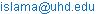(A. M. Islam)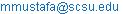(M. Mustafa)(M. Rahman)

Anisul M. Islam, Muhammad Mustafa, Matiur Rahman. Global Capital Mobility: Some New Empirical Evidence. Journal of World Economic Research. Special Issue: The Globalization and Economic Structure Changes. Vol. 4, No. 5-1, 2015, pp. 1-7. doi: 10.11648/j.jwer.s.2015040501.11

Abstract: Using Saving-Investment relationship as indirect evidence of global capital mobility, this paper empirically examines the capital mobility hypothesis using new data for forty developing countries. The paper utilizes annual data over 1960-2013 period, the longest time period of 54 years for as many developing countries ever used with a panel sample size of 2,160 (40 x 54) annual observations, the longest time periods and largest cross-sections ever used previously. For this study, panel regression analysis was used to estimate the relationship and then use the relationship to test some hypothesis regarding the capital mobility. The study finds evidence of partial capital mobility among the sample developing countries, and the degree of capital mobility was found to be stronger than that originally found by Feldstein and Horioka.

Keywords: Savings, Investment, Global Capital Mobility, Panel Regression

Contents

1. Introduction

The world has been witnessing increased globalization over the years, particularly since the fall of the Berlin Wall and the collapse of the Iron Curtain in 1989. This increased global integration is expected to lead to increased flow of goods, services, technology and resources including capital and financial resources. Of course, some of those may be more mobile than others, such as goods mobility might be higher than labor mobility, for example. Similarly, capital is expected to be more mobile than labor and capital mobility is also expected to have increased over the years in response to increased globalization and resulting deeper global integration. At the same time, it is also expected that capital mobility may be stronger among developed countries than developing countries to the extent that the financial markets in the former are likely to be open to capital mobility than the latter. This is, perhaps, due at least partly to higher level capital controls and public interventions in various forms to safeguard national economies from sudden and unexpected external negative financial shocks.

In light of this expectation, this study empirically examines the degree of global capital mobility across a group of 40 developing countries spanning the period from 1960 to 2013 for a sample period of 54 years, the longest time period ever used in previous studies. To analyze the issue of international capital mobility, we applied an indirect quantitative approach using domestic savings-investment correlation as a measure of international capital mobility. This approach is more convenient and applicable to all countries due to easier availability of reliable data.

The remainder of the paper is presented as follows. The section II presents a brief review of literature, while section III develops the empirical model along with hypothesis development. Section IV discusses the data followed by empirical results in section V. Section VI presents some concluding remarks.

2. A Brief Review of Literature

This approach was first applied in the seminal paper by Feldstein and Horioka (1980) using annual data on savings and investment rates for 16 OECD countries from 1960 through 1974 for cross-correlation. They hypothesized that domestic saving and investment rates are unrelated with perfect international capital mobility since it enables each country’s savings to respond to global investment opportunities while investment in each country is financed by accessing the global savings pool.

Surprisingly, Feldstein-Horioka (1980) concluded that the financial markets of 16 OECD countries were poorly integrated in light of the correlation coefficient ranging between 0.87 and 0.91 despite the presumption of their significant intra-financial globalization. The intuition is straightforward: if capital is perfectly mobile, domestic savings and investment should not be correlated (correlation should be low or non-existent indicating freer capital mobility), and capital should move freely to the place where the rate of return is the highest. Their observed results of high correlation indicated a quite low degree of financial integration, despite a large volume of international capital movements, domestic savings and investment across OECD countries, with estimated cross-correlation coefficient being close to one. The resulting Feldstein-Horioka puzzle opened the way for a large number of subsequent empirical studies analyzing the relationships between domestic savings and domestic investment. However, the puzzle seems to resist time, and appears to be even more at odds with current facts, when conventional wisdom strongly believes that international capital markets are being highly integrated (e.g., Van Wincoop, 2001).

The existing body of empirical literature on this important topic is vast, deep and expansive. So, a full coverage of the related literature is not provided in this paper. The counterintuitive finding of the Feldstein-Horioka (1980) running out of sync with the prior expectation of near-complete international capital mobility in the OECD countries sparked numerous subsequent studies (e.g., Apergis and Tsoumas, 2009; De Wet and Van Eyden, 2005; ), particularly in an era when concerted efforts were geared towards the enhancement of global capital market integration. Feldstein (1983) on 17 OECD countries using more expanded dataset also confirmed the existence of this "home-biased" investment climate.

Frankel et al. (1986) in their study, using data from 14 developed and 50 developing countries, found that savings and investment were highly correlated and shared a long-run equilibrium relationship, except in a few less developed countries. Similarly, Murphy (1984), Obstfeld (1986) and Wong (1990) found evidences for fair degree of association between domestic investment and savings for various less industrialized and developing countries. However, the results of their studies deviated from Feldstein-Horioka puzzle as the estimated correlation coefficients were lower in periods before the mid-1970s (period of relatively low capital mobility) than afterwards, when capital mobility was supposed to have increased.

There are many other studies that exist in this area, which were not covered here due to time and space limitations. These other studies, like those reported here, had used different sample of countries, covered different time periods, and used different methodologies. Not surprisingly, due to above differences, the results reported were generally mixed, some finding higher degree of capital mobility than others, begging for further studies to get better insights into the capital mobility hypothesis.

3. Empirical Model and Hypothesis Development

The finding of Feldstein-Horioka (1980) ran contrary to economic theory. Obsfeld and Rogoff (2000) termed it as "the mother of all puzzles". This paper seeks to revisit the "puzzle" by utilizing the panel regression analysis along with some hypothesis tests of capital mobility based on coefficient restrictions as discussed below.

Feldstein and Horioka (1980) examined the cross-sectional correlation between savings rate and investment rate by testing the following basic simple linear equation: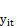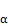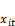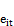(1)

Where, y=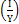represents the ratio (%) of investment over Gross Domestic Product (GDP), that is the investment rate. Similarly, x =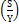is the ratio (%) of savings over GDP, can be interpreted as the savings rate. Index i stands for the considered country and index t stands for annual time periods,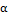is the common intercept term, and e is the random error term with standard i.i.d. properties. Now, adding country-specific fixed effects by γi in equation (1), we obtain the following extended basic linear model as follows=+ γi + 𝛽+(2)

Equation (2) is our basic empirically estimable model. In this model,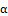is the common intercept term, β is the common slope parameter, γi shows the possibility of country-specific fixed effects reflecting country differences in institutional, cultural, legal, social, and economic characteristics and systems? Please note that this model can also be estimated based country-specific random effects in contrast to fixed effects. Further, we will conduct a hypothesis test related to examining whether random effects model or the fixed effect model is more appropriate as discussed below under Hypothesis#1. All different models will then be estimated using panel regression analysis with appropriate correction for multicollinearity, heteroskedasticty and autocorrelation.

Most important coefficient for this study is the slope parameter β, which has been the focus of substantial literature and is of primary importance in this paper for testing various hypotheses related to global capital mobility as elaborated below. In this model, lower the value of the slope coefficient 𝛽, the higher is the degree of capital mobility with 𝛽=0 as reflecting perfect capital mobility. This is because a lower value of 𝛽 indicates that most domestic investments are not dependent on or financed from domestic savings, rather these are financed from anywhere in the world, reflecting higher degree of capital mobility. In contrast, higher the value of the slope coefficient 𝛽, the lower is the degree of capital mobility with 𝛽=1 as reflecting no (zero) capital mobility. If 𝛽 lies between 0 (zero) to 1 (unity), which is more likely in the imperfect real world, the world will display partial or imperfect capital mobility with higher degree of capital mobility being indicated by the lower value of 𝛽. We will conduct two hypothesis tests based on coefficient restrictions on the slope coefficient 𝛽 for testing perfect versus no capital mobility as discussed under Hypothesis#2 and Hypothesis#3 below.

For further analysis, and as indicated above, we will conduct the following three hypothesis tests based on coefficient restrictions as follows:

Hypothesis#1: Testing for Random Effects versus Fixed Effects Model, the null and alternative hypotheses are as follows:

Ho: Random effects model is more appropriate

H1: Fixed effects model is more appropriate

Hypothesis#2: For perfect capital mobility, the null and alternative hypotheses on the slope coefficient are as follows:

Ho: 𝛽 = 0

H1: 𝛽 ≠ 0

Hypothesis#3: For no (zero) capital mobility, the null and alternative hypotheses on the slope coefficient are as follows:

Ho: 𝛽 = 1

H1: 𝛽 ≠ 1

4. The Data

Annual data for these developing countries for the above sample period are obtained from the World Bank’s World Development Indicators data base (World Bank 2015). The Panel data as a combination of cross-sectional i= 1, 2, 40 countries (cross-sections) and time series t= 1960, 1961, 2013 (54 years) with a total of 2,160 (=40 x 54) annual observations are used in this study. It is to be noted here that this sample involves longer time periods with more countries in the sample with a very large number of annual observations than utilized before. This provides a convenient way to study phenomenon where a statistically adequate number of cross-sectional and time series observations are not obtainable. This augments quality and quantity of data. Otherwise, it would be impossible to use only one of these two dimensions for meaningful analyses (Gujarati, 2003). This study provides an example of such situation where incorporating observations on the variables over successive time periods allows to expand the informational content of the data. Furthermore, since the length of the time series is relatively small compared to the number of cross-sectional observations, the effects of autocorrelation are small if not negligible. Panel data estimation models include the constant coefficient (pooled), the fixed effects and the random effects regression models.

5. Empirical Results

5.1. Model Selection and Analysis

Table 1 below provides the panel regression results of our basic model (equation (1)) and the extended basic model (equation (2)) with country-specific fixed effects. This table reports four different estimations based on the previous two model equations which are designated by Eq (1) through Eq (4) on the top row of this table. In this table, Eq (1) represents the basic model without country-specific fixed or random effects and without correcting for autocorrelation. Eq (2) represents the estimation of the basic extended model with country-specific fixed effects but without correcting for autocorrelation. Eq (3) reports the estimation results for the basic extended model with country-specific random effects without correcting for autocorrelation. Eq (3) is estimated to compare and conduct hypothesis test of whether random effects or the fixed effects model is more appropriate for the sample data. Finally, Eq (4) is estimated as the chosen model from the previous three to be re-estimated with correction for autocorrelation as the final basic extended model with country-specific fixed effects. Please note that the coefficients γi related to the country-specific fixed effects are not been reported in Table 1 to save space. There are forty country-specific fixed effects coefficients, one for each country, some being positive and some being negative, are not reported, but could be made available from the author upon request.

To select the best model for final interpretations and conclusions, we needed to compare all these four estimated regression equations. A comparison of all four Eq (i)’s, it is evident that in all cases, both the intercept and slope coefficients are highly statistically significant at better than 1% level of significance (probability values are zeros), R2’s and adj-R2’s are reasonable but Eq (4) has the highest R2 and Adj-R2 of about 80% . Further, the F-statistics are highly significant at better than 1% in all four versions. However, if we compare the D-W value, the first three versions suffer from significant positive autocorrelation while Eq (4) is free from autocorrelation as it re-estimates the fixed effect model (Eq (2), the basic extended model with country-specific fixed-effects ) with correction for autocorrelation. In this version, the AR(1) coefficient is highly statistically significant (at better than 1% level) and this correction has resulted in the D-W statistic of 2.01 indicating free of first-order autocorrelation. Based on the above comparison, it is clear that Eq (4) gives the best estimate for the model coefficients and would be focused on in making further discussion.

Table 1. Panel Regression Estimates-Four Versions of Two Models.

 Eq (1) Eq (2) Eq (3) Eq (4) Basic Model (No Fixed Effects) Extended Basic Model (Fixed Effects) Extended Basic Model (Random Effects) Extended Basic Model (Fixed Effects) with AR(1) Intercept () 14.10*** (0.00) 16.32*** (0.00) 16.01*** (0.00) 16.90*** (0.00) Slope (𝛽 ) 0.40*** (0.00) 0.28*** (0.00) 0.30*** (0.00) 0.27*** (0.00) γi NA NRa NA NRa R2 0.32 0.48 0.16 0.81 Adj-R2 0.32 0.47 0.16 0.80 F-Statistic 1038.70*** (0.00) 48.16*** (0.00) 397.38*** (0.00) 211.77*** (0.00) AIC 6.63 6.42 ----- 5.40 BIC 6.64 6.52 ----- 5.52 HQ 6.63 6.46 ----- 5.44 DW 0.33 0.41 0.40 2.01 AR(1) ----- ----- ----- 0.78***(0.00) Cross-sections 40 40 40 40 Time Periods 54 54 54 54 N 2160 2160 2160 2120

Notes: (1) Values in parentheses below the coefficients are probability values; (2) *** significant at 1% level; *** significant at 5% level; * significant at 10% level; (3) AIC: Akaike Information Criterion; BIC: Baysian Information criterion; HQ: Hannan-Quin criterion; DW: Durbin-Watson Criterion; N: Total sample size; (4) NA: Not applicable; (5) NRa: Not Reported; Forty country-specific fixed effects coefficients, one for each country, some positive and some negative, are not reported to save space. These are available from the author upon request.

5.2. Hypothesis Tests

Table 2. Hypothesis#1: Hauseman Test for Random vs. Fixed effects.

 Hypothesis#1: Ho: Random effects model is appropriate; H1: Fixed effects model is appropriate Test Summary Chi-Square Statistic Chi-Square Degree of Freedom Probability ----- Cross-section Random 17.22 1 0.00 ----- Cross-section random versus fixed effects test comparisons Slope Coefficient Fixed Random Var (diff.) Probability 𝛽 (Slope) 0.279936 0.296671 0.000016 0.0000

Dependent Variable: Investment rate (y); Explanatory variable: savings rate (x).

One question is why we have chosen Eq (2), the fixed effect model, to be re-estimated with correction for autocorrelation, but not Eq (3), the random effect model. That decision was based on the Hauseman test result of Hypothesis#1 given in Table 2. The bottom panel of this table shows that the 𝛽 coefficient is slightly higher than that of the fixed effects model and the difference is not that significant. In the top panel, it is shown that the random effects model with a Chi-square value of 17.22 with one degree of freedom and zero probability value, the null hypothesis (random effect model is more appropriate) is clearly rejected in favor of the alternative hypothesis that the fixed effects model is more appropriate for this sample data. So, Eq (4) was the result of choosing to re-estimating Eq (2) instead of Eq (3) to correct for autocorrelation.

We now turn to further interpretation of the magnitude of the slope coefficient, which is of primary importance in this study to examine the degree of capital mobility among nations, along with the two hypothesis tests reported earlier, i.e. testing Hypothesis#2 and the Hypothesis#3. With respect to the magnitude of the slope coefficients reported in Table 1, Eq (1) has the highest coefficient of 0.40, but this is not the best estimation version as discussed earlier. The remaining three versions have very similar coefficients, varying from 0.28 in Eq (2) to 0.30 in Eq (3) and 0.27 in Eq (4), the chosen best estimation. Given the chosen value of 0.27, it is clear that the coefficient is much closer to zero than to unity, indicating that the degree of capital mobility is quite strong among the sample developing countries. Further, this coefficient is much lower than that of 0.87 that was reported by Feldstein-Horioka (1980) for the 16 OECD countries.

Further, based on the coefficient of the chosen version (Eq (4)), we derived the 90% and 95% confidence intervals and these are reported in Table 3. The 95% confidence interval shows that the slope coefficient can vary within the range of 0.25 to 0.31, a very narrow range indeed around our chosen coefficient of 0.27.

Table 3. Confidence intervals based on the Chosen Version (Eq (4)).

 Coefficient Confidence Intervals Date: 05/30/15 Time: 16:24 Sample: 1960 2013 Included observations: 2160 90% CI 95% CI Variable Coefficient Low High Low High Intercept 16.32636 15.80759 16.84513 15.70812 16.94459 𝛽 (Slope) 0.279936 0.254652 0.305221 0.249804 0.310069

Table 4. Testing Hypothesis#2 of Perfect capital Mobility.

 Three Tests of Coefficient Restrictions Hypothesis#2: Ho: 𝛽 = 1; H1: 𝛽 ≠ 1 Test Statistic Value df Probability t-statistic -46.86313 2119 0.0000 F-statistic 2196.153 (1, 2119) 0.0000 Chi-square 2196.153 1 0.0000 Null Hypothesis: 𝛽 =1 Null Hypothesis Summary: Normalized Restriction (= 0) Value Std. Err. -1 + C(2) -0.720064 0.015365 Restrictions are linear in coefficients.

Table 5. Testing Hypothesis#3 of Zero (No) capital Mobility.

 Three tests of Coefficient restrictions Hypothesis#3: Ho: 𝛽 = 0; H1: 𝛽 ≠ 0 Test Statistic Value df Probability t-statistic 18.21879 2119 0.0000 F-statistic 331.9245 (1, 2119) 0.0000 Chi-square 331.9245 1 0.0000 Null Hypothesis: 𝛽 = 0 Null Hypothesis Summary: Normalized Restriction (= 0) Value Std. Err. C(2) 0.279936 0.015365 Restrictions are linear in coefficients.

To test for the hypothesis of perfect capital mobility, we turn to the test result for Hypothesis#2 based three different tests, the t-test, the Wald Wald-F test and the Chi-Square test of coefficient restrictions (Gujarati 2003) as reported in Table 4. All three tests clearly show that the null hypothesis of perfect capital mobility is rejected at better than 1% level of significance with probability values of zeros in all three tests. It is thus clear as expected that perfect capital mobility does not exist in the world as of today even with high degree of globalization and global integration among nations.

To test for the hypothesis of zero (no) capital mobility, we turn to the test result for Hypothesis#3 based on three different tests, the t-test, the Wald Wald-F test and the Chi-Square test of coefficient restrictions (Gujarati 2003) as reported in Table 5. All three tests clearly show that the null hypothesis of zero capital mobility is rejected at better than 1% level of significance with probability values of zeros in all three tests. It is thus clear as expected that zero or no capital mobility does not exist in the world, given today’s high degree of globalization and global integration among nations.

5.3. Diagnostic Tests

To examine whether the residuals from the chosen model (Eq (4)) satisfies important statistical properties such as being well-behaved and satisfy i.i.d. properties, we conducted some diagnostic tests for the residuals obtained from the chosen equation Eq (4) reported in Table 1. A test for normality was conducted and reported in Figure 1. The results show that the residuals show slight negative Skewness but excessive Kurtosis (much in excess of k = 12.89 > 3 for normal distribution). With the Jarque-Bera statistic of 8642.13 with probability value of zero, the null hypothesis of normality is clearly rejected. However, rejection of normal distribution for the residuals is not an i.i.d. requirement for unbiased estimators in regression analysis.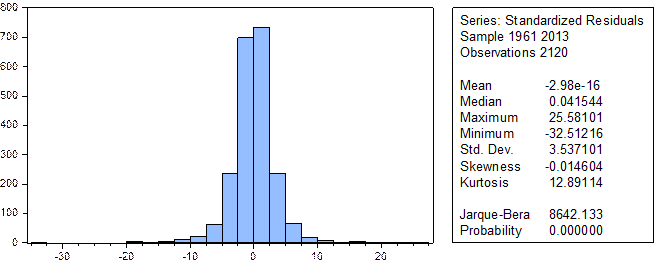Figure 1. Residual Normality Test.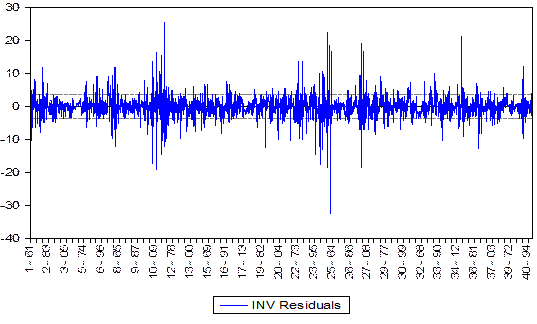Figure 2. Residual Plot.

We also conducted tests for residual auto-correlation and found the presence of first-order autocorrelation in the residuals. We then corrected the autocorrelation issue and estimated the model with correction for first order autocorrelation and the autocorrelation corrected results were used to be the our best estimated equation reported as Eq (4) in Table 1. Further, we plotted the residuals as shown in Figure 2. This figure clearly shows that the residuals do not show any regular pattern or trend and hence appear to be stationary and free of autocorrelation.

6. Concluding Remarks

In a world with high degree of globalization and global integration, it is expected that capital mobility among nations would be strong. We utilized the original Feldstein-Horioka (1980) framework to examine the degree of capital mobility among nations along with some hypothesis tests based on coefficient restrictions as developed and discussed earlier in the paper.

Our results are based on the chosen Eq (4) which provides estimates for the basic extended model with country-specific fixed effects and with correction for first order autocorrelation as reported in table 1. This result is followed by a number of further tests such as estimation of the confidence intervals, hypothesis testing for random versus fixed effects model, and two further hypothesis tests of perfect versus zero capital mobility hypotheses. Given the estimated chosen slope coefficient value of 0.27 in table 1 with the narrow 95% confidence interval range of 0.25 to 0.31 (Table 3), and the tests results of Hypothesis#2 rejecting the null hypothesis of perfect capital mobility and that of Hypothesis#3 rejecting zero capital mobility, we conclude that the world, at least in our sample 40 developing countries, clearly displays a great deal of capital mobility among these countries, albeit indicating imperfect and partial but not perfect capital mobility.

Further, the degree of capital mobility found and reported in this paper is much higher even among the sample of 40 developing countries in our panel data sample compared to what was found by Feldstein-Horioka (1980) even among the more advanced 16 OECD countries. This result suggests that although much quantitative and qualitative restrictions on capital mobility exists in today’s many developing countries, the higher degree of globalization and global integration has resulted in much more capital mobility than in the past decades. Given the relatively larger number of countries with a very long time period from 1960 to 2013 and a panel sample size of 2,160, the estimated results seem to be quite robust and hence highly reliable. In addition, we have conducted some diagnostic checks on the residuals obtained from the chosen equation, the results show that the residuals are free of autocorrelation and are generally well-behaved.

In terms of policy implication, it can be suggested that the countries which still lag behind significantly in terms of trade and financial globalization need to catch up and reform their economies to enable them to attract foreign capital to finance their domestic investment needs such as major industrial and infrastructure projects. If greater degree of foreign capital can be attracted for domestic investment, these economies would likely experience higher economic growth and better standard of living for their citizens as done by China for example in its post-1978 reform period.

References

1. Apergis, N., and Tsoumas, C. (2009). "A survey on the Feldstein-Horioka puzzle: What has been done and where we stand", Research in Economics, 63: 64-76.
2. De Wet, A.H., and Van Eyden, R. (2005). "Capital mobility in Sub-Saharan Africa: A panel data approach," South African Journal of Economics, 73, 1-22.
3. Feldstein, M. (1983). "Domestic saving and international capital movements in the long-run and the short-run", European Economic Review, 21: 129-151.
4. Feldstein, M., and Horioka, C. (1980). "Domestic saving and international capital flow", The Economic Journal, 90: 314-329.
5. Frankel, J., Dooley, J., and Matheison, D. (1986). "International capital mobility in developing countries Vs industrial countries: What do savings-investment correlation tell us, NBER Working Paper, 2043.
6. Gujarati, D. (2003). "Basic econometrics", Fourth Edition, McGraw- Hill.
7. Murphy, R. (1984). "Capital mobility and relationship between saving and investment rates in OECD countries", Journal of International Money and Finance, 327-332.
8. Obstfeld. M. (1986). "Capital mobility in the world economy: Theory and Measurement", Carnegie-Rochester Conference Series on Public Policy, 24: 55-104.
9. Obstfeld. M., and Rogoff, K. (1995). "The intertermporal approach to the current account", NBER Working Paper, 4893.
10. Van Wincoop, E. (2001). "Intranational versus international saving-investment comovements", In G. Hess and E. Van Wincoop (eds.), International Macroeconomics, Cambridge: Cambridge University Press, 1-43.
11. Wong, D. (1990). "What do saving-investment relationships tell us about capital mobility?", Journal of International Money and Finance, 9: 60-74.
12. World Bank (2015), World Development Indicators, World Bank website, Washington D.C. USA, available at www.worldbank.org.

 Contents 1. 2. 3. 4. 5. 5.1. 5.2. 5.3. 6.
Article ToolsAbstractPDF(286K)